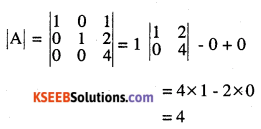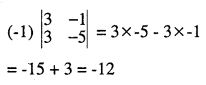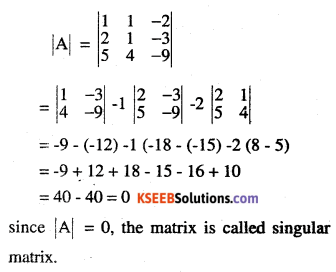2nd PUC Maths Question Bank Chapter 4 Determinants Ex 4.1

Students can Download Maths Chapter 4 Determinants Ex 4.1 Questions and Answers, Notes Pdf, 2nd PUC Maths Question Bank with Answers helps you to revise the complete Karnataka State Board Syllabus and score more marks in your examinations.

Karnataka 2nd PUC Maths Question Bank Chapter 4 Determinants Ex 4.1

2nd PUC Maths Determinants NCERT Text Book Questions and Answers Ex 4.1

Evaluate the determinations

Question 1.
$$\left|\begin{array}{rr}{2} & {4} \\{-5} & {-1}\end{array}\right|$$
2 x (-1) -4x (-5)
= -2 + 20 = 18

Question 2.
(i)
$$\left|\begin{array}{rr}{\cos \theta} & {-\sin \theta} \\{\sin \theta} & {\cos \theta}\end{array}\right|$$
Cos θ x Cos θ – sin θ x sin θ
= cos2 θ + sin2 θ = 1

(ii)
$$\left|\begin{array}{cc}{\mathbf{x}^{2}-\mathbf{x}+\mathbf{1}} & {\mathbf{x}-\mathbf{1}} \\{\mathbf{x}+\mathbf{1}} & {\mathbf{x}+\mathbf{1}}\end{array}\right|$$
(x2 – x + 1) (x + 1) – (x – 1) (x + 1)
= x3 + 1 – (x2 – 1)
= x3 – x2 + 2Question 3.
$$\text { If } \mathbf{A}=\left[\begin{array}{ll}{1} & {2} \\{4} & {2}\end{array}\right]$$,then show that |2A| = 4 |A|Question 4.
$$\text { If } \mathbf{A}=\left[\begin{array}{lll}{\mathbf{1}} & {\mathbf{0}} & {\mathbf{1}} \\{\mathbf{0}} & {\mathbf{1}} & {\mathbf{2}} \\{\mathbf{0}} & {\mathbf{0}} & {\mathbf{4}}\end{array}\right]$$ then show that |3A| = 27 |A|Question 5.
Evaluate the determinant
(i)
$$\left|\begin{array}{rrr}{3} & {-4} & {5} \\{1} & {1} & {-2} \\{2} & {3} &{1}\end{array}\right|$$
Expanding along R2 (Second Row where two elements are zeroes)(ii)
$$\left|\begin{array}{rrr}{3} & {-4} & {5} \\{1} & {1} & {-2} \\{2} & {3} & {1}\end{array}\right|$$
Expanding along R1(iii)
$$\left|\begin{array}{ccc}{0} & {1} & {2} \\{-1} & {0} & {-3} \\{-2} & {3} & {0}\end{array}\right|$$
Expanding along R1(iv)
$$\left|\begin{array}{ccc}{2} & {-1} & {-2} \\{0} & {2} & {-1} \\{3} & {-5} &{0}\end{array}\right|$$
Expanding along R3Question 6.
$$\text { If } A=\left[\begin{array}{ccc}{1} & {1} & {-2} \\{2} & {1} & {-3} \\{5} & {4} & {-9}\end{array}\right] \text { find }|A|$$Question 7.
find X if
(i)
$$\left|\begin{array}{cc}{2} & {4} \\{5} & {1}\end{array}\right|=\left|\begin{array}{cc}{2 x} & {4} \\{6} & {x}\end{array}\right|$$
2 x 1 -4 x 5 = 2 × x × -4 × 6
2 – 20 = 2x2 – 24 – 18
= 2x2 – 24
2x2 = 6,
x2 = 3
$$x=\pm \sqrt{3}$$

(ii)
$$\left|\begin{array}{cc}{2} & {3} \\{4} & {5}\end{array}\right|=\left|\begin{array}{cc}{x} & {3} \\{2 x} & {5}\end{array}\right|$$
2 x 5 -3 x 4=5 x x – 6x
10 – 12  = 5x – 6x
-2       =-x
x = 2Question 8.
$$\text { If }\left|\begin{array}{cc}{x} & {2} \\{18} {x}\end{array}\right|=\left|\begin{array}{cc}{6} & {2} \\{18} & {6}\end{array}\right|$$
(A) 6
(B) ± 6
(C) -6
(D) 0